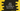# Java program to check if a number is a buzz number or not## Introduction :

In this java buzz programming tutorial, we will learn how to check if a number is buzz or not.

A number is called a buzz number if it is :

1. Divisible by 7 or

2. Ends with 7

So, to find out if a number is buzz will not be tough. The only thing we need to check is the above two conditions.

Our program will first ask the user to enter a number. It will then check the above two conditions to find out if it is buzz or not. And finally, it will print it out.

### Java buzz number program :

``````import java.util.Scanner;

public class Example {
public static void main(String[] args) {
//1
int no;
Scanner sc;

//2
String isBuzzNo = " is a buzz number as";
String strDivisibleBy7 = " it is divisible by 7";
String strEndsWith7 = " ends with 7";

//3
sc = new Scanner(System.in);
System.out.println("Enter a number you want to check : ");

//4
no = sc.nextInt();

//5
if (no % 10 == 7) {
//6
if (no % 7 == 0) {
System.out.println(no + isBuzzNo + strDivisibleBy7 + " and" + strEndsWith7);
} else {
System.out.println(no + isBuzzNo + " it" + strEndsWith7);
}
} else if (no % 7 == 0) {
//7
System.out.println(no + isBuzzNo + strDivisibleBy7);
} else {
//8
System.out.println(no + " is not a buzz number");
}
}
}
``````

### Explanation of the above java buzz number program :

The commented numbers in the above program denote the step numbers below :

1. Define one integer variable no to store the user input value and one Scanner variable sc to read all user inputs.

2. In this step, we are creating three strings. These strings are used for customizing the final result output to the user.

3. Create the Scanner variable sc and ask the user to enter a number that is required to check. Read the number using the scanner variable and store it in the no variable.

4. We are using if-elseif-else condition to check if the number is buzz or not. The first if statement is to check if the number ends with 7 or not. We are using % to check if the number is ending with 7 or not. All the numbers that satisfy this condition is a buzz number.

5. Inside, check again if the number is divisible by 7 or not. If yes, it means that the number is ending with 7 and also divisible by 7.Print out the message as it is a buzz number and it is divisible by 7 and also ending with 7. If the if condition fails, print out that it is a buzz number and it is ending with 7.

Actually the if-else statement inside the if statement of step 5 is not required. We are using these statements to print the message with more detail to the user.

1. If step 6 fails, check if the number is divisible by 7 or not. If yes, print it out as the number is a buzz number and also it is divisible by 7.

2. Finally, if all if and else-if cases failed, print out that it is not a buzz number.

### Sample Output :

``````Enter a number you want to check :
19
19 is not a buzz number

Enter a number you want to check :
707
707 is a buzz number as it is divisible by 7 and ends with 7

Enter a number you want to check :
17
17 is a buzz number as it ends with 7

Enter a number you want to check :
21
21 is a buzz number as it is divisible by 7
``````

### Conclusion :

This java buzz number tutorial explained to you on how to find if a number is a buzz or not. Try to run the program we have explained above and drop one comment below if you have any queries.